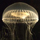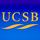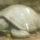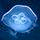## General Question# How do you go about adding polynomials with fractions in parantheses?

Asked by emeraldisles (1949) January 5th, 2012

The problem is( 1/16x^2+1/8)+(¼x^2–3/10x-½). The answer is supposed to be 5/16x^2–3/10x-3/8. All I can figure out is that the numbers/fractions all need to be changed into least common denominator. Have no idea how to get to the next step to get this answer?Help.

Observing members: 0Composing members: 0Response moderated (Writing Standards)No they don’t need to be to the LCD – that rule is for adding, not multiplying.

(A +B) (C+D+E) = AC+AD+AE+BC+BD+BE = 1/64X^2…

Charles (4823)“Great Answer” (0) Flag as…Okay, lets do an easier one:

(⅓x + 2/5) + (¾x + ½)

Take the “x terms.” These are ⅓x and ¾x Lets get common denominators:
⅓x = 4/12x
¾x = 9/12x
4/12x + 9/12x = (4/12 + 9/12)x = 13/12x

Take the numbers. These are 2/5 and ½. Lets get common denominators:
2/5 = 4/10
½ = 5/10
2/5 + ½ = 4/10 + 5/10 = 9/10

So, the total is
13/12x + 9/10

————————————————————————————————————————————————————-

Okay, lets make it a bit harder.
(1/5x^2 + ⅓x + 2/5) + (⅔x^2 + ¾x + ½)

Take the “x^2 terms.” These are 1/5x^2 and ⅔x^2 Lets get common denominators:
1/5x^2 = 3/15x^2
⅔x^2 = 10/15x^2
3/15x^2 + 10/15x^2 = (3/15 + 10/15)x^2 = 13/15x^2

Take the “x terms.” These are ⅓x and ¾x Lets get common denominators:
⅓x = 4/12x
¾x = 9/12x
4/12x + 9/12x = (4/12 + 9/12)x = 13/12x

Take the numbers. These are 2/5 and ½. Lets get common denominators:
2/5 = 4/10
½ = 5/10
4/10 + 5/10 = 9/10

So, the total is
13/15x^2 + 13/12x + 9/10

———————————————————————————————————————-

roundsquare (5522)“Great Answer” (3) Flag as…disregard the parenthesies, and only add like terms, coefficients with the same variables, don’t add 3a to 4b or 5a^2 to 6a, order of operations

s321scba (67)“Great Answer” (0) Flag as…or